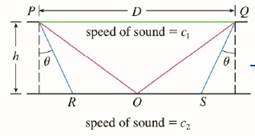Chapter 3.P, Problem 19P

Chapter
Section
Textbook Problem

# The speeds of sound c 1 in an upper layer and c 2 in a lower layer of rock and the thickness h of the upper layer can be determined by seismic exploration if the speed of sound in the lower layer is greater than the speed in the upper layer. A dynamite charge is detonated at a point P and the transmitted signals are recorded at a point Q, which is a distance D from P. The first signal to arrive at Q travels along the surface and takes T 1 seconds. The next signal travels from P to a point R, from R to S in the lower layer, and then to Q, taking T 2 seconds. The third signal is reflected off the lower layer at the midpoint O of RS and takes T 1 seconds to reach Q. (See the figure.)(a) Express T 1 T 2 , and T 3 in terms of D , h , c 1 , c 2 , and θ .(b) Show that T 2 is a minimum when sin θ = c 1 / c 2 (c) Suppose that D = 1 k m , T 1 = 0.26 s , T 2 = 0.32 s , and T 3 = 0.34 s Find c 1 , c 2 and h .Note: Geophysicists use this technique when studying the structure of the earth’s crust, whether searching for oil or examining fault lines.

To determine

a)

To express:

T1,T2  and T3 in terms of D, h,c1,c2 and θ

Explanation

1) Concept:

The function is minimum when f'x<0  and maximum when f'x>0.

Power rule of differentiation ddxxn=nxn-1

Chain rule ddxfxgx=fxddxgx+gxddxf(x)

time=distancespeed

2) Calculation:

time=distancespeed

T1=Dc1

T2=2(PR)c1+RSc

To determine

b)

To show:

T2 is minimum when sin θ=c1c2

To determine

c)

To find:

The values of h,c1,c2 when T1=026s, T2=0.325s,  T3=0.34s, D=1km.

### Still sussing out bartleby?

Check out a sample textbook solution.

See a sample solution

#### The Solution to Your Study Problems

Bartleby provides explanations to thousands of textbook problems written by our experts, many with advanced degrees!

Get Started

#### Use sets toshow that 06=0.

Mathematical Excursions (MindTap Course List)

#### In Problems 7-34, perform the indicated operations and simplify. 30.

Mathematical Applications for the Management, Life, and Social Sciences

#### Sometimes, Always, or Never: If f(c) = 0, then c is a critical number.

Study Guide for Stewart's Single Variable Calculus: Early Transcendentals, 8th